Gradient descent is a popular optimisation technique, and forms the basis of many learning algorithms. Perhaps most notably, Gradient Descent is used when training many types of neural networks. There are a few variants of this technique, but the simplest version is Batch Gradient Descent. In this post, we’ll introduce this algorithm, and then go over 3 key advantages and disadvantages of this method. Finally, we’ll use 2 illustrative examples in Python to explore some of the aforementioned characteristics of Batch Gradient Descent. Anyone interested in using this approach, to train their model, should be aware of these points.What is Batch Gradient Descent? 3 Pros and Cons – image by author

## What is Batch Gradient Descent?

Generally speaking, gradient descent is an optimisation algorithm that attempts to find the best set of model parameters \vec{w} (otherwise termed ‘weights‘) for a given problem. This is done by first selecting a loss function \ell, by which the effectiveness of the model can be measured.  As we improve the values of the weights, the model will perform better on the problem at hand. In other words, as \vec{w} is optimised, \ell will decrease. Therefore, we wish to find the set of model parameters that yields the minimum value of \ell

Some key points to make note of:

1. The approach we will take is iterative in nature, meaning we will repeat the stages of our algorithm a number of times as we ‘step’ towards our goal. This will not be an analytical solution.
2. We need to define a loss function \ell for our problem, that is also a differentiable function of the model parameters \vec{w}. If our problem, or choice of model, does not facilitate this, we cannot use gradient descent.

To move towards the minimum value of \ell, we will compute the derivative with respect to the model parameters, \partial\ell / \partial w. Since the derivative points towards the maximum value of \ell in weight space, we will take a step in the opposite direction. The update rule is therefore defined by:

\vec{w’} = \vec{w} – \alpha\frac{\partial \ell}{\partial w}    (1)

where \vec{w’} is the updated set of model parameters after a single step in our algorithm. The learning rate \alpha is a hyperparameter, and controls how large the steps will be. Typical values for \alpha range from 0.1 to 0.001.

We will repeat the update defined in (1) a number of times, until either the magnitude of the update falls below a tolerance threshold \tau, or the maximum number repetitions has been made. At each step, we move towards the loss minimum in weight space. Figure 1 illustrates this scenario: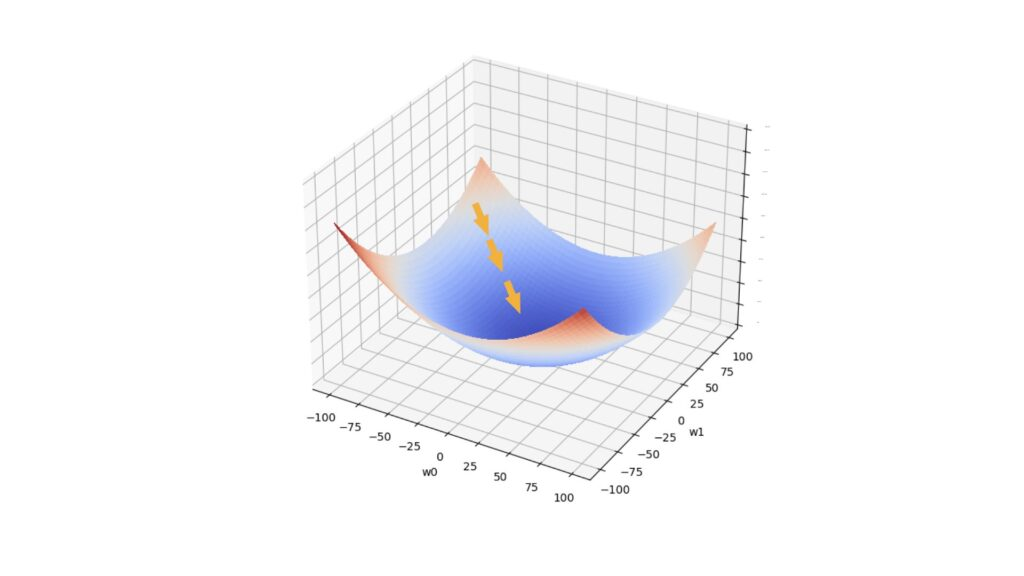Figure 1: One possible path taken during the gradient descent algorithm. The horizontal axes represent 2 model parameters, whereas the vertical axis represents the loss value. The arrows indicate the movement taken during gradient descent, from a location of high loss to one with low loss.

With Batch Gradient Descent, we use all the available training data to compute the model parameter updates, at each iteration. This algorithm consists of the following:

1. Choose values for the learning rate \alpha, tolerance threshold \tau, and number of epochs. The latter defines the maximum number of steps we will take in our procedure.
2. Initialise the values of the model parameters \vec{w}.
3. for i = 1…\text{number of epochs}:
• compute the average derivative \partial\ell / \partial w over all the training data.
• update the model parameters according to equation (1).
• check if the magnitude of our update, \alpha\frac{\partial \ell}{\partial w}, falls below \tau. If so, exit the loop.

## 3 Pros of Batch Gradient Descent

There are a number of advantages to choosing Batch Gradient Descent, over other optimisation methods. Let’s outline 3 of the most important here.

### I) Steady and Accurate Convergence to a Minimum

As all the training data is considered when making a single update to the model parameters, Batch Gradient Descent will normally yield a direct path to a minimum. Figure 1 illustrates this scenario. This means little time will be spent moving in incorrect directions in weight space. If the learning rate \alpha is not too big, this also means Batch Gradient Descent will yield a very accurate estimate of the minimum (when compared to other gradient descent methods).

### II) Easy Implementation

The Batch Gradient Descent algorithm is quite simple to implement in code, when compared to other variations. The number of hyperparameters introduced is kept to a minimum, and the implementation can be fully vectorised. We will work through an implementation of this algorithm later on in this article.

### III) Excellent for Convex Functions

Batch Gradient Descent works very well for convex functions with smaller-sized datasets. In such a scenario, all derivatives \partial\ell / \partial w point to the single global minimum, and there will be no issue loading all the training data into memory for processing. An example convex loss function is illustrated in Figure 1.

## 3 Cons of Batch Gradient Descent

There are also some downsides to using Batch Gradient Descent in a given machine learning project. Let’s outline 3 of the most relevant.

### I) Unpractical for Larger Datasets

As all the training data is used to compute each step in our algorithm, this approach is very computationally expensive. All the training data must be loaded into memory. For Big Data, this simply isn’t feasible.

### II) Slow to Converge

Related to the first point; since all the training data is used to compute each step, this puts strain on the processing resources we have available. The algorithm may only slowly converge towards a minimum for larger datasets.

### III) Difficult to Escape Local Minima

For non-convex loss functions, many local minima will be present in weight space. This scenario is illustrated in Figure 3 below. Batch Gradient Descent is not well designed to explore the parameter space: instead it tends to lead towards a direct path to the nearest minimum. As such, this algorithm can get stuck at a local minimum in these situations.

## Python Examples

I would now like to make some of the concepts discussed more tangible. Let’s work through an implementation of Batch Gradient Descent in Python, and test it with two different functions on a simple toy dataset. As a model to train, we will be making use of a simple linear regression.

### MSE Loss Function

The mean squared error (MSE) is a common loss function used in regression problems. This is due to its simplicity, as well as to the fact that it is differentiable. Mathematically this is given by:

\ell = \frac{1}{2N}\sum^N_i (y_{i,pred}-y_{i,true})^2    (2)

where y_{i,true} are the true target values, and y_{i,pred} are the model predictions. For our purposes here, the latter quantity is given by: y_{i,pred} = w_0 + w_1x_i, where w_0 is the bias, x_i is a single predictor feature, and w_1 is the corresponding weight for this feature. Note that \ell is a convex function of the model parameters.  Figure 2 visualises this function below.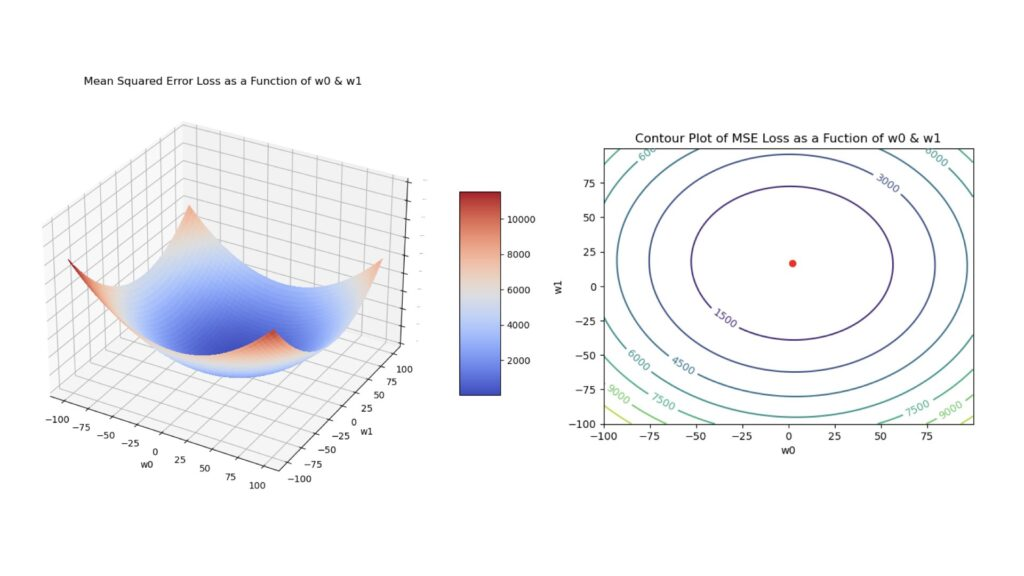Figure 2: Surface plot (left) and contour plot (right) of the MSE loss. These plots were generated using a simple linear regression to produce predictions, and each plot was made as a function of the model parameters \vec{w} = [w_0, w_1]. The red dot in the contour plot indicates the location of the global minimum.

### Trigonometric Function

Let’s also define a more complicated function, that will produce a surface with many local minima and maxima. This function is defined by:

\ell = \frac{1}{2N}\sum^N_i \theta_i^2sin\left(\frac{\pi \theta_i}{50}\right)    (3)

where \theta_i = (y_{i,pred}-y_{i,true}), and all the other parameters are the same as was defined for the MSE loss. Note that in this case, \ell is non-convex. Figure 3 illustrates this function below: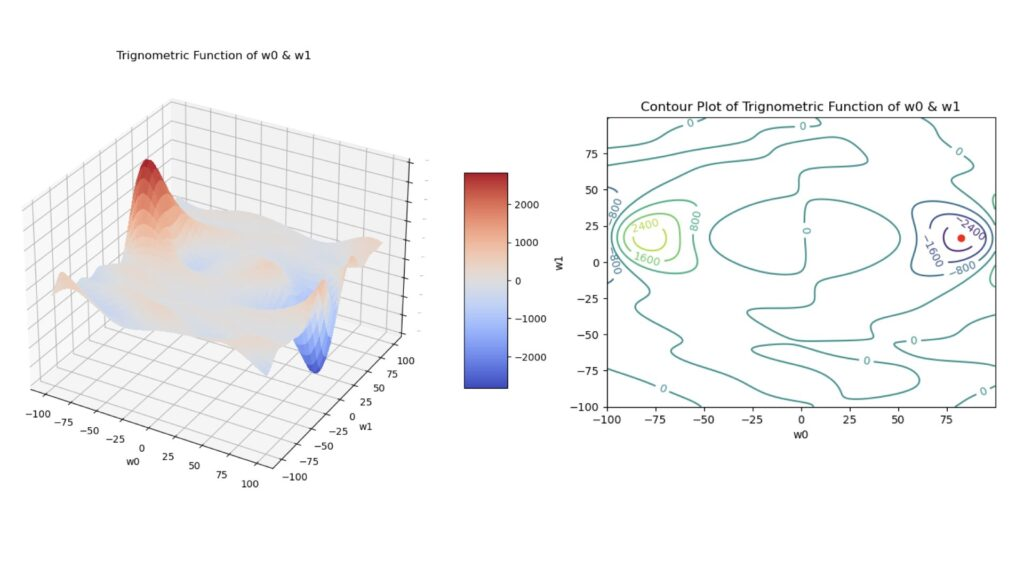Figure 3: Surface plot (left) and contour plot (right) of the trigonometric function defined in (3). These plots were generated using a simple linear regression to produce predictions, and each plot was made as a function of the model parameters \vec{w} = [w_0, w_1]. The red dot in the contour plot indicates the location of the global minimum.

Note that (3) is not a loss function used in machine learning, as the minimum of \ell does not coincide with our desired state (i.e. y_{i,pred}=y_{i,true}). We will make use of it here simply because it provides a highly complex surface, with local maxima and minima, in weight space.

We can now put together a single Python function that will encompass the Batch Gradient Descent algorithm. This code will make use of the numpy package.

# imports
import numpy as np
import matplotlib.pyplot as plt
from matplotlib import cm
from matplotlib.ticker import LinearLocator
from typing import Callable, Tuple, Union
from sklearn.datasets import make_regression
from sklearn.metrics import mean_squared_error, mean_absolute_error

y: np.array,
model: Callable,
Dloss: Callable,
lr: float=0.1,
epochs: int=500,
tol: float=1e-4,
verbose: bool=False) -> Tuple[np.array, list]:
"""
Function to compute batch gradient descent for the provided training data using the input model & loss

Inputs:
Inputs:
X       -> predictor values
y       -> true target values
model   -> function to compute model predictions
Dloss   -> function to compute the derivate of the loss function in weight space
lr      -> learning rate
epochs  -> number of epochs to train
tol     -> tolerance for early stopping
verbose -> boolean to determine if extra information is printed out

Output
tuple containing a numpy array with the obtimised weights & list of the traversed path in weight space
"""
# initialise weights
w_max  = 100.0
w      = w_max*(np.random.rand(X.shape,1) - 0.5)
w_path = []

# cycle through epochs and update weights using batch gradient descent
for _ in range(epochs):
# store current weight values
w_path.append(w.tolist())
# make predictions
yp = model(X,w)
# compute the weights update & check tolerance
dw = lr*Dloss(X,y,yp)
w -= dw
if np.linalg.norm(dw) < tol:
break

if verbose:
yp = model(X,w)
print(f"Error metrics: MSE = {mean_squared_error(y,yp):.2f}, MAE = {mean_absolute_error(y,yp):.2f}")
print(f"Final weights: {float(w):.2f}, {float(w):.2f}")

return (w, w_path)
• The training data is composed of an array of predictor features X, and associated true target values y.
• The input argument model is a function used to compute predictions at each iteration of the algorithm.
• Dloss is another function, that computes the derivative of the (loss) function we are using.
• The learning rate lr was termed \alpha in (1). This controls the magnitude of the update step at each iteration.
• The epochs argument defines the maximum number of times we pass through the entire set of training data. This is also the same as the number of iterations in our algorithm.
• The tolerance tol, referred to as \tau above, sets a lower bound for the size of our updates. The algorithm stops early if the magnitude of the update falls below this value.
• The boolean argument verbose controls if performance metrics, and the final weights, are printed out once the algorithm finishes.

Let’s now write the remaining functions we’ll need for our tests. This will include a function for generating predictions from a linear regression model, as well as calculating the derivatives of (2) and (3):

def model(X: np.array, w: np.array) -> np.array:
"""
Function to compute a linear model

Inputs:
X -> predictor values
w -> weights

Output:
numpy array of predicted target values
"""
return np.matmul(X,w)

def mse_gradient(X: np.array, y: np.array, yp: np.array) -> np.array:
"""
Function to compute the derivative of the mse loss

Inputs:
X  -> predictor values
y  -> true target values
yp -> predicted target values

Output
numpy array containing the mse gradient
"""
return 1/(X.shape)*np.sum(X*(yp-y).reshape(-1,1),axis=0).reshape(-1,1)

def trig_gradient(X: np.array, y: np.array, yp: np.array) -> np.array:
"""
Function to compute the derivative of the trignometric function

Inputs:
X  -> predictor values
y  -> true target values
yp -> predicted target values

Output
numpy array containing the trignometric gradient
"""
theta = yp - y
return 1/(X.shape)*np.sum((theta*X)*(2*np.sin(np.pi*theta/50)
+(np.pi*theta/50)*np.cos(np.pi*theta/50)),axis=0).reshape(-1,1)

### Create a Toy Dataset

I will make use of the make_regression function, from scikit-learn, to produce some data:

# make a simple regression dataset
X, y, coef = make_regression(n_samples=1000, n_features=1, noise=5, bias=2, coef=True, random_state=42)

Let’s visualise the data we’ve just created:

# plot the generated data
plt.scatter(X,y)
plt.xlabel('X')
plt.ylabel('y')
plt.title('Generated Dataset')
plt.show()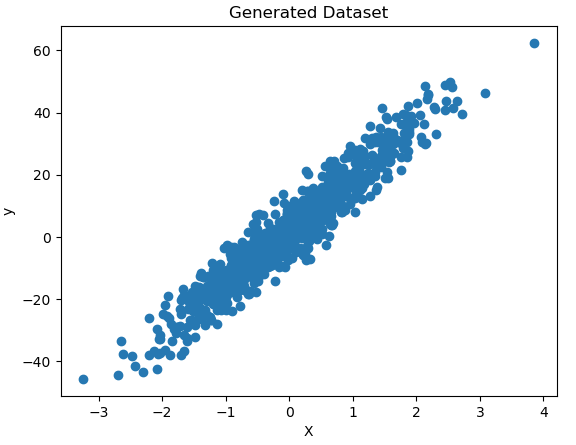Figure 4: Generated toy dataset

Before testing our implementation on these data, let’s first add a column of 1’s to  X to account for the bias parameter w_0. Afterwards, we can also make note of the true value of w_1:

# add bias term
b = np.ones((X.shape,1))
X = np.concatenate((b,X),axis=1)

# reshape true labels
y = y.reshape(-1,1)

# what is the model parameter?
coef
array(16.74825823)

Note that the true value of w_0 was set to 2.0 when we made the call to make_regression (see the bias input argument).

### Batch Gradient Descent with MSE Loss

We can now test out our implementation using the MSE loss function, with 800 epochs and verbose set to True. All other parameters are set to their default values:

w, w_path = batch_gradient_descent(X, y, model, mse_gradient, epochs=800, verbose=True)
Error metrics: MSE = 24.50, MAE = 3.95
Final weights: 2.02, 16.69

The final weight values look quite good! Indeed they are very close to the true values of (2.0, 16.75).

I ran this code 3 separate times, to record the paths traversed by our implementation. Let’s take a look at how those trials turned out: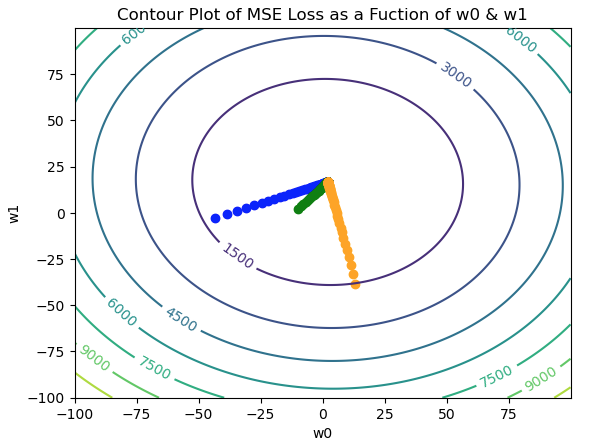Figure 5: Contour plot for the MSE loss function, with 3 separate paths traversed in weight space. The paths are indicated in blue, green, and orange, and all converge to the global minimum near the center of the figure.

It is apparent in Figure 5 that each trial converges to the same point, regardless of the initial (w_0,w_1) position. Note the paths traversed are also linear and direct.

### Batch Gradient Descent with Trigonometric Function

Let’s now test out our implementation using the trigonometric function, described in equation (3). Here I’ll set the learning rate \alpha to 0.01, and request 1000 epochs:

w, w_path = batch_gradient_descent(X, y, model, trig_gradient, lr=0.01, epochs=1000, verbose=True)
Error metrics: MSE = 1279.44, MAE = 35.43
Final weights: -33.40, 16.68

We can see the resulting weights are not nearly as close to the true values, as was seen previously. Unsurprisingly, the quality of the final generated predictions, reflected through the reported error metrics, is also not as good.

Like before, I ran this experiment 3 times and recorded the paths traversed in weight space. Let’s look at these results: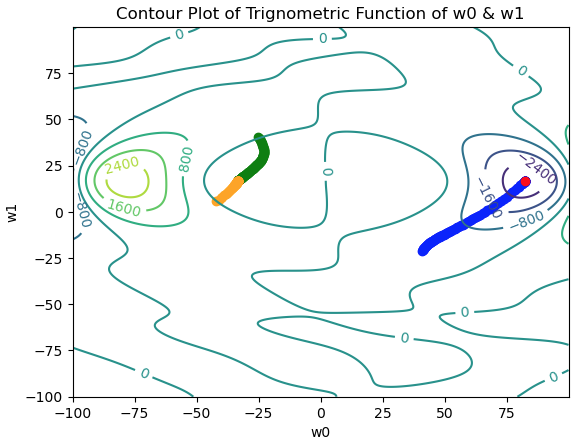Figure 6: Contour plot for the trigonometric function described in (3), with 3 separate paths traversed in weight space. The paths are indicated in blue, green, and orange. Note the lack of convergence; there is no single point all the paths converge to. The true global minimum is indicated in red.

We can see the 3 trials move towards minima within their vicinity, however the positions settled upon are highly dependent on the initial starting positions in weight space. The green and orange trials converge on a broad local minimum, whereas the blue trial finds the global minimum. Note each path outlines a smooth trajectory from start to end positions.

## Final Remarks

In this post we have covered:

1. What is the Batch Gradient Descent algorithm, and what it is used for.
2. 3 Pros and Cons for using this variant of gradient descent.
3. How to implement Batch Gradient Descent in Python from scratch.
4. Explored how well does Batch Gradient Descent do when applied to convex and non-convex functions.

I hope you enjoyed this article, and gained some value from it. If you would like to take a closer look at the code presented here, please take a look at my GitHub. If you have any questions or suggestions, please feel free to add a comment below. Your input is greatly appreciated.

### Related Posts

5 1 vote
Article Rating
Subscribe
Notify of